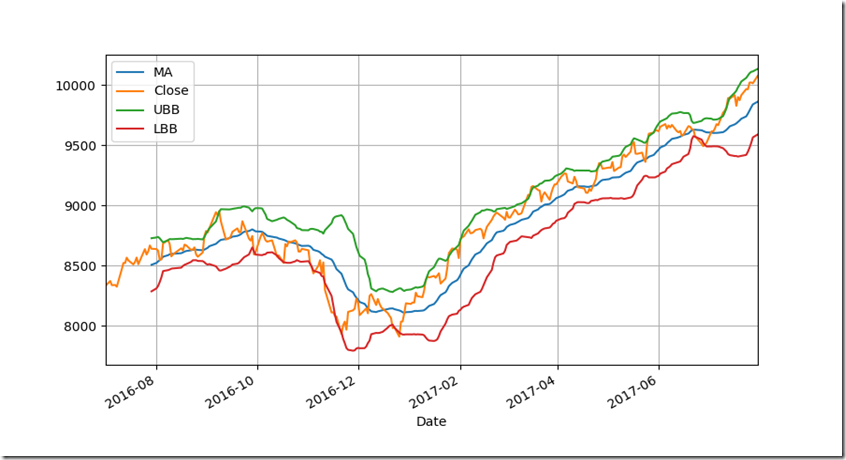### Calculate Pre-Determined Prices of Various Stocks with the help of Bollinger Bands in Python.Bollinger Bands is referred as Volatility Bands it is mainly used to measure the Volatility of Price Movement it was introduced by John Bollinger.

Bollinger Bands is a Trade Mark and Registered in the name of Bollinger Bands to Measure the Volatility as it Increases and Decreases.

Bollinger Bands are Calculated with the help of Moving Average and Standard Deviation in which it will be Above and Below the Moving Average Price .

Above graph or line is referred as Upper Band and Below one is referred as Lower Band in Between Moving Average price.

Moving Average Can be Calculated can be Calculate by taking Averages and then we Should Calculate the Standard Deviation which can be attained with the help of moving average

Then the Upper Band is Calculated and Visualized by having moving average and then it is added with standard deviation multiplied with the factor of 2.

The Lower Band is Calculated and Visualized by having moving average and then it is subtracted with standard deviation multiplied with the factor of 2.

All of the Explanation can be understood with the help of figure given above.From the Figure we Can see that It forms a W-Bottoms and then suddenly a huge raise in the price of that particular stock which can be easily measured and if a M-Bottoms is formed we can understand Vice Versa and then after if the Moving Average Price Crosses Upper Band it is measured as Over Bought Levels and if it crosses the Lower Bands it Can be Measured as Over Sold Levels then if the price is squeezed in between then we can say that a Buy Level is about to form and Vice Versa.

Bollinger Bands Does Lot of Contraction and Relaxation from which we can use that thing and have better understanding and can realise the Pre-Determined Stock Price Movement for further Calculation and Visualization please Follow the Video and the Python Code Input is Available Below which you Can Try it by Yourself.

Compute Pre-Determined Prices of Various Stocks with the help of Bollinger Bands in Python Part-1

Compute Pre-Determined Prices of Various Stocks with the help of Bollinger Bands in Python Part-2

``````
#import the required packaging and modules
import pandas as pd
import matplotlib.pyplot as plt
import fix_yahoo_finance

#compute the bollinger bands
def BBANDS(data,ndays):
MA=data.Close.rolling(n).mean()
SD=data.Close.rolling(n).std()
data['MA']=MA
data['UBB']=MA+(2*SD)
data['LBB']=MA-(2*SD)
return data
#retrieve the stock data from yahoo finance
data=pdr.get_data_yahoo('^NSEI',start='2016-07-01',end='2017-08-01')
data=pd.DataFrame(data)

#compute the Bollinger Bands with 20 day moving average
n=20
NBB=BBANDS(data,n)
print(NBB)

#create the plot with Close,Moving Average,Upper band,Lower band
pd.concat([NBB.MA,NBB.Close,NBB.UBB,NBB.LBB],axis=1).plot(figsize=(9,5),grid=True)
plt.plot()
plt.show()
#if want to save those graph in PNG format please comment it out above line i.e plt.show()
plt.savefig('BBANDS.png')
``````
``` ```

--End of The Code Input--